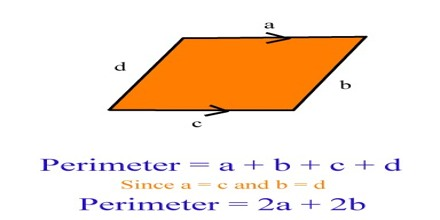Calculating the Perimeter of a Parallelogram

Perimeter of parallelogram means the total distance around the outside of a parallelogram. The perimeter of a parallelogram is also said to be the distance at its outer surface or the length of all its edges. It is the sum of lengths of its sides. The perimeter of a shape is calculated by the measuring its edges.

The perimeter of a parallelogram is the distance around the outside of the parallelogram. A parallelogram has four sides with opposite sides being congruent. The formula for finding the perimeter is Side A + Side B + Side A + Side B.This could also be stated as: (2 x Side A) + (2 x Side B) or [2 x (Side A + Side B)].

Formula; Perimeter = c (base x side) = 2 (w + h)

where:

• w is the base length of the parallelogram
• h is the side length

Example: Find the perimeter of a parallelogram whose adjacent sides are 10 cm and 24 cm.

Solution: Let a = 10 cm and b = 24 cm

Perimeter = 2 (a + b)

= 2 (10 + 24)

= 2 x 34

= 68

Perimeter of a parallelogram is 68 cm.